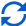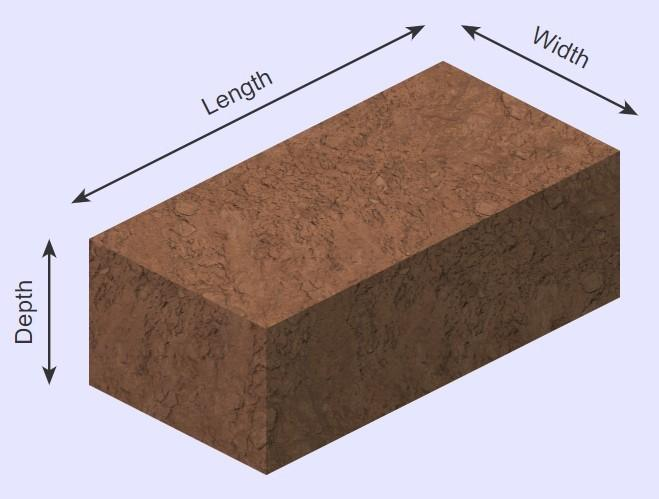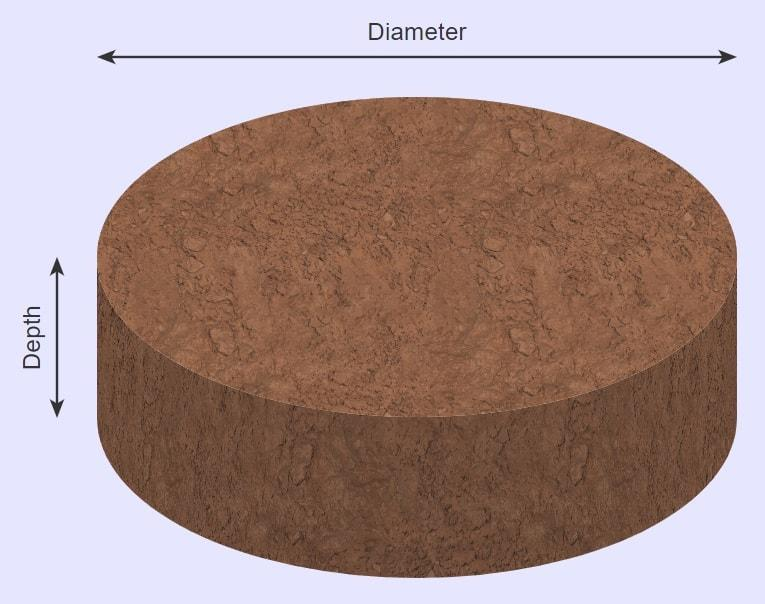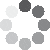Enter parameters Rectangular area
Enter parameters
LENGTH, WIDTH & DEPTH
Length:
ft
• inches (in)
• feet (ft)
• yards (yd)
• centimeters (cm)
• meters (m)
Width:
ft
• inches (in)
• feet (ft)
• yards (yd)
• centimeters (cm)
• meters (m)
Depth:
in
• inches (in)
• feet (ft)
• yards (yd)
• centimeters (cm)
• meters (m)
Bag size:
ft³
• cubic inches (in³)
• cubic feet (ft³)
• cubic yards (yd³)
• cubic centimeters (cm³)
• cubic meters ()
Density:
100 - Soil lbs/ft³
• Soil - 100 lbs/ft³
• Custom
Price per unit of mass:
t
• pounds (lb)
• US short tons (t)
• imperial-long tons (long t)
• kilograms (kg)
Price per unit of volume:
yd³
• cubic yards (yd³)
• cubic feet (ft³)
• cubic inches (in³)
• cubic centimeters (cm³)
• cubic meters ()Result
Area:
0
ft²
• square feet (ft²)
• square inches (in²)
• square yards (yd²)
• square miles (mi²)
• acres (ac)
• square centimeters (cm²)
• square meters ()
Volume:
0
yd³
• cubic yards (yd³)
• cubic feet (ft³)
• cubic inches (in³)
• cubic centimeters (cm³)
• cubic meters ()
Weight:
0
t
• pounds (lb)
• US short tons (t)
• imperial-long tons (long t)
• kilograms (kg)
Cost:
$Bags: 0 Enter parameters Diameter: ft • inches (in) • feet (ft) • yards (yd) • centimeters (cm) • meters (m) Depth: in • inches (in) • feet (ft) • yards (yd) • centimeters (cm) • meters (m) Bag size: ft³ • cubic inches (in³) • cubic feet (ft³) • cubic yards (yd³) • cubic centimeters (cm³) • cubic meters () Density: 100 - Soil lbs/ft³ • Soil - 100 lbs/ft³ • Custom Price per unit of mass: t • pounds (lb) • US short tons (t) • imperial-long tons (long t) • kilograms (kg) Price per unit of volume: yd³ • cubic yards (yd³) • cubic feet (ft³) • cubic inches (in³) • cubic centimeters (cm³) • cubic meters ()Result Area: 0 ft² • square feet (ft²) • square inches (in²) • square yards (yd²) • square miles (mi²) • acres (ac) • square centimeters (cm²) • square meters () Volume: 0 yd³ • cubic yards (yd³) • cubic feet (ft³) • cubic inches (in³) • cubic centimeters (cm³) • cubic meters () Weight: 0 t • pounds (lb) • US short tons (t) • imperial-long tons (long t) • kilograms (kg) Cost:$
Bags:
0(No Ratings Yet)Loading...
Similar Calculators:
Real-time graphics. Make the calculations and see the changes.
Real-time graphics. Make the calculations and see the changes.
Embed
Similar Calculators:Estimating how much soil your next landscaping project will require is no mean feat.

You need to take account of the shape you need to fill, the density of the soil and how deep you want the garden bed to be.

Guessing this by eye will often lead to over-ordering bags of soil!

That’s why we built our soil calculator. 🏗️

Alongside a little preparation, it can save you time and money with your next gardening project.

We will also detail the formulae it uses alongside some fully worked examples.

Contents:

## Soil calculator formula

The soil calculator first works out the area of the garden bed you will be looking to fill with soil.

It then works out the volume of soil required based on the depth of the garden bed.

It estimates this using the following formulae:

### Rectangular AreaThe area and volume of a rectangular garden bed is calculated by:

$$Area = Length \times Width$$

$$Volume = Area \times Depth$$

### Circular AreaThe area and volume of a circular garden bed is calculated by:

$$Area = \pi × \biggl( {Diameter \over 2} \biggr) ^2$$

$$Volume = Area \times Depth$$

If you know the density of the soil you are using, then the calculator can work out the total weight of the soil required using the formulae:

$$Weight = Volume \times Density$$

Note that standard soil has a density of 100 lb/ft³.

But what if you’re using a custom soil aggregate and you don’t know it’s density?

Well, you can work out the density of the soil aggregate using the formulae:

$$Density = {Total\,mass\,of\,soil \over Total\,volume\,of\,soil}$$

## How to find the cost of the project

If you know the price per unit mass (e.g. cost per pound) or cost per unit volume (e.g. cost per cubic foot), then the calculator can work out the total cost of the gardening project:

$$Cost = Price\,per\,unit\,mass \times Weight$$

or

$$Cost = Price\,per\,unit\,volume \times Volume$$

If you don’t know the price per unit mass/volume, you can calculate it using the formulae:

$$Price\,per\,unit\,mass = {Total\,soil\,cost \over Total\,soil\,weight}$$

$$Price\,per\,unit\,volume = {Total\,soil\,cost \over Total\,soil\,volume}$$

Confused?

Keep reading for two fully worked examples starting with different initial criteria.

Otherwise, enter your measurements and values into our online soil calculator to get your next gardening project off to an efficient start!

## Example calculations

• Rectangular bed area with standard soil

Let’s say that I have a raised rectangular garden bed that I want to fill with standard soil (100 lb/ft³).

The measurements of the raised bed are 4 feet in length and 2 feet in width.

I want to ensure equal coverage of soil to a depth of 6 inches.

I can purchase soil at a cost of $100 per US short ton. I want to know how much soil I will need to completely fill my raised garden bed, and how much this will cost. I enter these measurements into the calculator which would do the following operations to work out the area and volume of the garden bed: $$Area = Length \times Width = 4\,ft \times 2\,ft = 8\,ft^2$$ $$Volume = Area \times Depth = 8\,ft^2 \times 6\,in = 4\,ft^3$$ Then, the calculator would work out the total weight of the soil: $$Weight = Volume \times Density = 4\,ft^3 \times 100\,lb/ft^3 = 0.2\,t$$ Finally, the calculator would work out the total cost of the soil: $$Cost = Price\,per\,unit\,mass \times Weight = 100\,/t \times 0.2\,t = 20$$ • Circular bed area with custom soil aggregate and unknown cost per unit volume For the second example imagine I have a raised circular garden bed which measures 10 feet in diameter. I want to fill it with a custom soil aggregate which I can purchase at a cost of$100 for 2 cubic yards, weighing 2000 pounds in total.

I want to ensure an average coverage of 4 inches.

I can therefore calculate its density (in pounds per cubic yard) and price per unit volume (in dollars per cubic yard):

$$Density = {Mass \over Volume} = {2000\,lb \over 2\,yd^3} = 1000\,lb/yd^3$$

$$Price\,per\,unit\,volume = {Total\,soil\,cost \over Total\,soil\,volume} = {100 \over 2\,yd^3} = 50\,/yd^3$$

Now, I simply enter the measurements into the calculator which does the following operations to work out the bed area and volume:

$$Area = \pi × \biggl( {Diameter \over 2} \biggr) ^2 = \pi × \biggl( {10\,ft \over 2} \biggr) ^2 = 78.54\,ft^2$$

$$Volume = Area \times Depth = 78.54\,ft^2 \times 4\,in = 26.18\,ft^3$$

Finally, the calculator works out the weight and cost of the raised circular bed based on the measurements calculated above:

$$Weight = Volume \times Density = 26.18\,ft^3 \times 1000\,lb/yd^3 = 0.485\,t$$

$$Cost = Price\,per\,unit\,volume \times Volume = 50\,/yd^3 \times 26.18\,ft^3 = 48.48$$

Finally, it’s likely that you are asking:

## What if I want to work with different units?

You might have noticed that we change between units in our examples above. ⬆️

For example, density is given in pounds per cubic yard and volume is given in cubic feet.

You don’t need to worry about changing between different units throughout the calculations.

Our online soil calculator takes care of this for you, saving you time and effort!

Simply select the correct units in the drop down areas and the calculator will do the following conversions for you:

$$1\,foot = 12\,inches = 0.33\,yards = 30.48\,centimeters = 0.3048\,meters$$

$$1\,US\,short\,ton = 2000\,pounds = 0.893\,imperial\,long\,ton = 907\,kg$$

## How many bags of soil do i need?

You might be wondering how many bags of soil you will need for your gardening project.

Well, this can easily be calculated if you know the weight or volume of a single bag of soil.

You can do this by using the formulae:

$$Number\,of\,bags\,required= {Weight\,of\,soil\,required \over Weight\,of\,a\,single\,bag\,of\,soil}$$

or

$$Number\,of\,bags\,required= {Volume\,of\,soil\,required \over Volume\,of\,a\,single\,bag\,of\,soil}$$

Let’s take the second worked example above, where we required 26.18 cubic feet of soil.

Let’s imagine that soil is sold in bags measuring 0.75 cubic feet.

Therefore:

$$Number\,of\,bags\,required= {Volume\,of\,soil\,required \over Volume\,of\,a\,single\,bag\,of\, soil} = {26.18\,ft^3 \over 0.75\,ft^3} = 34.9 \rightarrow 35\,bags$$

It’s that simple! 😉

Embed this calculator on your site!Add live graphics
Copied to clipboard! Preview